## Excentral Triangle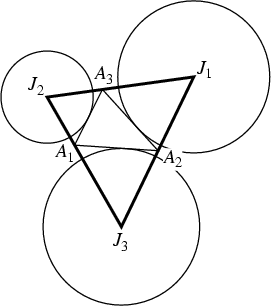The Triangle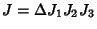with Vertices corresponding to the Excenters of a given Triangle, also called the Tritangent Triangle.

Beginning with an arbitrary Triangle, find the excentral triangle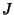. Then find the excentral triangle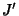of that Triangle, and so on. Then the resulting Triangle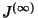approaches an Equilateral Triangle.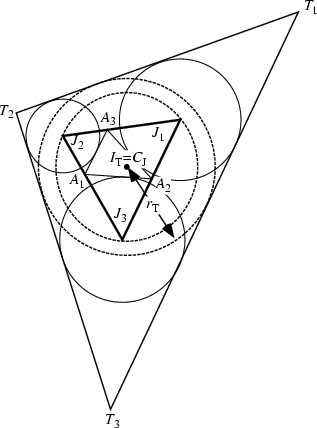Call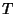the Triangle tangent externally to the Excircles of. Then the Incenter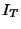of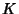coincides with the Circumcenter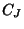of Triangle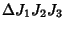, where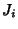are the Excenters of. The Inradius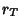of the Incircle ofis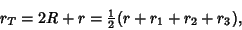where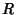is the Circumradius of,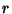is the Inradius, and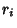are the Exradii (Johnson 1929, p. 192).